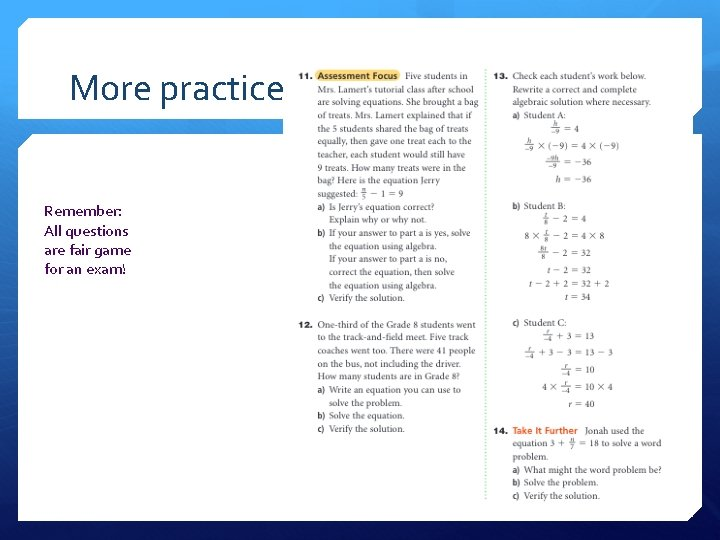# Solving Equations Involving Fractions Math 8 Linear Equations

• Slides: 9Solving Equations Involving Fractions Math 8 – Linear Equations & GraphingClassroom Challenge Problem A carnival comes to Calgary. Mia has some tickets for the midway. She shares her tickets with two friends so that they each get nine tickets. How many tickets did Mia start with?Classroom Challenge Problem Let t represent the starting number of tickets 9 = t/3 The starting tickets divided by the 3 people equals nine tickets each. Cross multiply 9 x 3 = t/3 x 3 t = 27 Verify: 9 = t/3 9 = 27/3Classroom Challenge Problem #2 School council sold T-shirts for charity. The council bought the t-shirts in boxes of 30. They added \$5 to the original price of each shirt and gave the profit to charity. What did they initially pay for one box of t-shirts if they sold each shirt for \$29?Classroom Challenge Problem #2 Write an equation to represent the problem and solve it. Let c represent the cost of each t-shirt box. Therefore each t-shirt costs c/30 \$5 was added to the cost of each t-shirt so now we have c/30 +5 Each shirt was sold for \$29 so the equation is: c/30 + 5 = 29Classroom Challenge Problem #2 Solve using algebra: c/30 + 5 = 29 c/30 + 5 – 5 = 29 – 5 c/30 = 24 c = 720 Therefore, the school council paid \$720 for each box.Practice Do questions: 3. a & b 4. c & d 5. All parts 6. All parts 8. a, b, & d 10.More practice Remember: All questions are fair game for an exam!Supplemental Workbook Do pages 145 -147 in your workbook. All is due: Monday, Feb. 26 th REMINDER: There is a high probability that we will have a quiz next week featuring: Modeling and solving two-step and one-step algebraic problems using tiles Solving algebraic problems concretely (without models) Verifying our answers